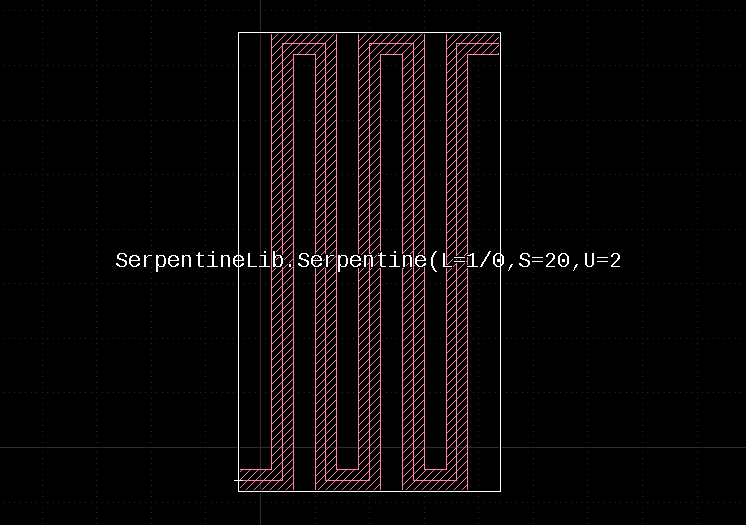Meander structure design

Hi,
I am trying to design a meander structure (trace). Is there any script or macro to help or ease such design?

With best regards,
Supratim

• Hi,

I had once created a PCell with such a code.

I'll share it here:

import pya
import math

"""
This Code implements a Serpentine PCell

It will generate a path starting from 0,0 and produce a serpentine this
way:

+->+  +->    ^
^  |  ^      |
|  |  |      |
|  |  |      | s
|  |  |      |
|  |  |      |
|  V  |      |
+->+  +->+      V

<-> u

The parameters are:
- l: the layer to use
- w: the width of the path
- n: the number of vertical paths
- u: see drawing above
- s: see drawing above

NOTE: using negative angles makes the Serpentine turn the other way

"""

class Serpentine(pya.PCellDeclarationHelper):
"""
The PCell declaration for the Serpentine
"""

def __init__(self):

# Important: initialize the super class
super(Serpentine, self).__init__()

# declare the parameters
self.param("l", self.TypeLayer, "Layer", default = pya.LayerInfo(1, 0))
self.param("n", self.TypeInt, "Number of points per full turn", default = 5)
self.param("w", self.TypeDouble, "The width", default = 1.0)
self.param("u", self.TypeDouble, "One turn's pitch", default = 2.0)
self.param("s", self.TypeDouble, "The turn's length", default = 20.0)

def display_text_impl(self):
# Provide a descriptive text for the cell
return "Serpentine(L=%s,S=%.12g,U=%.12g" % (str(self.l), self.s, self.u)

def produce_impl(self):

# This is the main part of the implementation: create the layout

# compute the Serpentine: generate a list of spine points for the path and then
# create the path

pts = []

x = 0.0
y = 0.0

for i in range(0, self.n):
pts.append(pya.DPoint(x, y))
x += self.u
pts.append(pya.DPoint(x, y))
if (i % 2) == 0:
y += self.s
else:
y -= self.s
pts.append(pya.DPoint(x, y))

# One last point to move to the end location
x += self.u
pts.append(pya.DPoint(x, y))

# create the shape
self.cell.shapes(self.l_layer).insert(pya.DPath(pts, self.w))

class SerpentineLib(pya.Library):
"""
The library where we will put the PCell into
"""

def __init__(self):

# Set the description
self.description = "Serpentine PCell Library"

# Create the PCell declarations
self.layout().register_pcell("Serpentine", Serpentine())

# Register us with the name "SerpentineLib".
self.register("SerpentineLib")

# Instantiate and register the library
SerpentineLib()

This is a sample image:Matthias

• Thank you very much, Matthias. It's really helpful. Btw, I'm a beginner with Klayout script. Do you know where can I find the documents about classes in "pya" package. e.g. I want to know all the parameters and methods in pya.PCellDeclarationHelper. Where can I find that?

thank you very much,
Zuyuan

• @ZuyuanTian I can offer the documentation which is online:

Matthias